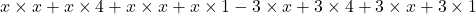## Please help. I will mark you as brainliest ! If x-3 is a factor of P(x)=x^3- 7x²+15x – 9, which of the following represents the

Question

If x-3 is a factor of P(x)=x^3- 7x²+15x – 9, which of the following represents the complete factorization for P(x)?
A. (x – 3)(x+4)(x+1)
B. (x – 3)(x+3)(x+1)
c. (x – 3)(x+3)(x-1)
D. (x – 3)(x – 3)(x-1)

in progress 0
6 months 2021-08-05T00:52:31+00:00 2 Answers 2 views 0

## Answers ( )